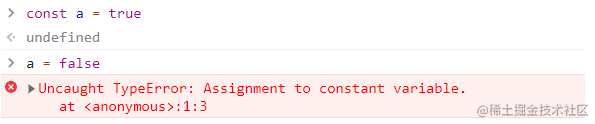# JavaScript 新特性应该这样回答## JavaScript 新特性

### 1 声明变量

ES6之前，声明变量只能使用var关键字。而现在，我们有几种不同的选择，而且功能都得到了增强

#### 1.1 const关键字#### 1.3 模板字符串

``````const name = 'xiaoming';

console('我的名字是' + name)；

``````const name = 'xiaoming';

console(`我的名字是 \${name}`);

### 2 创建函数

#### 2.1 函数声明

``````// 声明
function fun() {}

// 调用
fun()

#### 2.2 函数表达式

``````// 声明
const fun = function() {}

// 调用
fun()

##### 传递参数

``````// 声明
const fun = function(num) {}

// 调用
fun(1)

##### 函数返回值

``````// 声明
const fun = function(num) {
return num + 1
}

// 调用
fun(1)

#### 2.3 默认参数

``````// 声明
const fun = function(num = 10) {
return num + 1
}

// 调用
fun()

#### 2.4 箭头函数

``````const fun = num => num + 1;

``````const fun = (num1, num2) => num1 + num2;

`````` const fun = (num1, num2) => {
...
return num1 + num2;
};

``````const per = (firstName, lastName) => {
first: firstName,
last: lastName,
}``````const per = (firstName, lastName) => ({
first: firstName,
last: lastName,
})

``````const tahoe = {
mountains: ['Freel', 'Tallac', 'Rubicon', 'Silver'],
print: function(delay = 1000) {
setTimeout(function() {
console.log(this.mountains.join(','));
}, delay);
}
}

``````console.log(this); // window``````const tahoe = {
mountains: ['Freel', 'Tallac', 'Rubicon', 'Silver'],
print: function(delay = 1000) {
setTimeout(() =>{
console.log(this.mountains.join(','));
}, delay);
}
}

tahoe.print();// Freel,Tallac,Rubicon,Silver

``````const tahoe = {
mountains: ['Freel', 'Tallac', 'Rubicon', 'Silver'],
print: (delay = 1000) => {
setTimeout(() =>{
console.log(this.mountains.join(','));
}, delay);
}
}

### 3 编译 JavaScript

``````const add = (x = 5, y = 10) => console.log(x + y);

``````"use strict";
var x =
argument.length <= 0 || argument === undefined ? 5 :argument;
var y =
argument.length <= 0 || argument === undefined ? 10 :argument;
return console.log(x + y);
}

Babel添加了“use strict”声明，在严格模式下运行代码。变量x和y通过arguments数组(你可能熟悉这个技术)模拟成默认参数。编译得到的JavaScript支持范围更广。

### 4 对象和数组

#### 4.1 析构对象

``````const sandwich = {
meat: "tuna",
chese: "swiss",
toppings: ["lettuce", "tomato", "mustard"]
};
const { bread, meat } = sandwich;
console.log(bread, meat); // dutch crunch tuna

``````const sandwich = {
meat: "tuna",
chese: "swiss",
toppings: ["lettuce", "tomato", "mustard"]
};
const { bread, meat } = sandwich;
meat = "turkey";

console.log(meat); // turkey

console.log(sandwich.bread, sandwich.meat); // dutch crunch tuna

``````const obj = {
firstName: 'aa',
lastName: 'bb'
};
const lordify = ({ firstName }) => {
console.log(`\${firstName} 是我的姓！`);
};

lordify(obj); // aa是我的姓！

``````const obj = {
firstName: 'aa',
lastName: 'bb'
like: {
one: '篮球'，
two: '足球'，
};
};
const lordify = ({ like: {one} }) => {
console.log(`\${one} 是我的爱好！`);
};

lordify(obj); // 篮球是我的爱好！

#### 4.2 析构数组

``````const [first] = ['1', '2', '3'];

console.log(first); // 1

``````const [, , third] = ['1', '2', '3'];

console.log(third); // 3

#### 4.3 对象字面量增强

``````const name = 'xiaoming';
const like = '篮球'；

const obj = {name, like};

console.log(obj); // {name: 'xiaoming', like: '篮球'}

``````// 旧方法
var skier = {
name: name,
sound: sound,
fun: function() {
console.log('123456');
}
};

// 新语法
// 旧方法
const skier = {
name,
sound,
fun() {
console.log('123456');
}
};

#### 4.4 展开运算符

``````const arr1 = ['xiaoming', 'xiaowang', 'xiaoli'];
const arr2 = ['小明', '小王', '小李'];

const arr3 = [...arr1, ...arr2];

console.log(arr3.join(',')); // xiaoming,xiaowang,xiaoli,小明,小王,小李

``````const arr1 =  ['xiaoming', 'xiaowang', 'xiaoli'];
const [last] = arr1.reverse();

console.log(last); // xiaoli
// 原数组被改变了
console.log(arr1); // ['xiaoli', 'xiaowang', 'xiaoming']

``````const arr1 =  ['xiaoming', 'xiaowang', 'xiaoli'];
const [last] = [...arr1].reverse();

console.log(last); // xiaoli
// 原数组没有被改变了
console.log(arr1); // ['xiaoming', 'xiaowang', 'xiaoli']

``````const arr2 = ['小明', '小王', '小李', '小傻瓜'];
const [first, ...others] = arr2;

console.log(others.join(',')); // 小王,小李,小傻瓜

``````function funArr(...args) {
let [first, ...arr] = args;
console.log(first);
console.log(arr);
}
funArr('1', '2', '3', '4');
// 1
// ['2', '3', '4']

``````const obj = {
name: 'xiaoming',
like: '篮球',
};

const work = '程序员';

const obj2 = {
...obj,
work
};

console.log(obj2); // {name: 'xiaoming', like: '篮球', work: '程序员'}